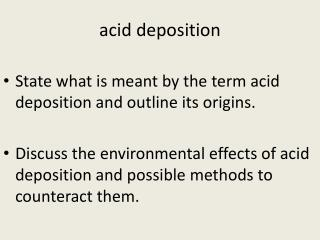DownloadDownload Presentationacid deposition

# acid deposition

Télécharger la présentation## acid deposition

- - - - - - - - - - - - - - - - - - - - - - - - - - - E N D - - - - - - - - - - - - - - - - - - - - - - - - - - -
##### Presentation Transcript

1. acid deposition • State what is meant by the term acid deposition and outline its origins. • Discuss the environmental effects of acid deposition and possible methods to counteract them.

2. rainwater normally acidic Write equations to show that rain water is normally acidic.

3. rainwater normally acidic • Rain is naturally slightly acidic because of the carbon dioxide dissolving into rain water to form carbonic acid according to the equation: CO2 (g) + H2O (l)  H2CO3 (aq) • Carbon acid is a weak acid that dissociates partially to produce H+ ions to make rain water slightly acidic. H2CO3 (aq)  HCO3- (aq) + H+(aq) Acidic rain is rain with a pH of less than 5.6 as it contains nitric acid and sulphuric acid.

4. acid deposition • Acid deposition refers to the process by which acid particles (acid solution particles or particles which can form acidic solutions i.e. H+(aq))leave the atmosphere as they are brought back down to earth e.g. on the ground, on trees, buildings, inside plants (e.g. stomata) or animals (e.g. your lungs). • There are two types of acid deposition: • wet deposition: acid rain, fog, sleet and snow – involves water. • dry deposition: gas molecules or acidic particles attached onto small airborne particles such as dust.

5. Nitric acid Write equations to show how nitric acid is formed in the atmosphere.

6. acid deposition: origins NO/NO2: formed as a result of high temperatures in internal combustion engines, i.e. cars and jet engines. 1. Production of nitrogen oxides: N2 (g) + O2 (g)  2NO (g) 2NO(g) + O2 (g)  2NO2 (g) 2. The following equations show two ways in which nitric (V) acid is formed in the atmosphere 2NO2(g) + H2O (l)  HNO3 (aq) + HNO2 (aq) (=nitrous acid) Or 4NO2(g) + 2H2O (l) + O2 (g) 4 HNO3 (aq)

7. Sulphuric acid • Equations!!

8. acid deposition: origins SO2/SO3: from burning of coal which contains sulphur and from smelting plants • Production of oxides: S (g) + O2 (g)  SO2 (g) 2SO2 (g) + O2 (g)  2SO3 (g) • Formation of atmospheric sulphuric (VI) acid and sulfurous acid SO3 (g) + H2O (l)  H2SO4 (aq) 2SO2 (g) + 2H2O (l) + O2 (g) 2H2SO4 (aq) SO2 (g) + H2O (l)  H2SO3(aq)

9. acid deposition

10. acid deposition

11. acid deposition: action • Cutting down emissions of nitrogen oxides by using catalytic converters, lean burn engines and thermal exhaust systems (lowers operating temperature). • Use of low sulphurfuels or remove sulphur before use. • Removal of sulphur oxides from exhaust fumes (factories, powr station stacks, incinerators) ; alkali scrubbers, limestone based fluidized bed. • Use of alternative sources of energy which do not involve fossil fuels and the production of very high temperatures. • Dealing with acid rain itself: neutralization of lakes using calcium carbonate or calcium oxide, e.g. CaO + H2SO4 (aq)  CaSO4 (s) + H2O (l)